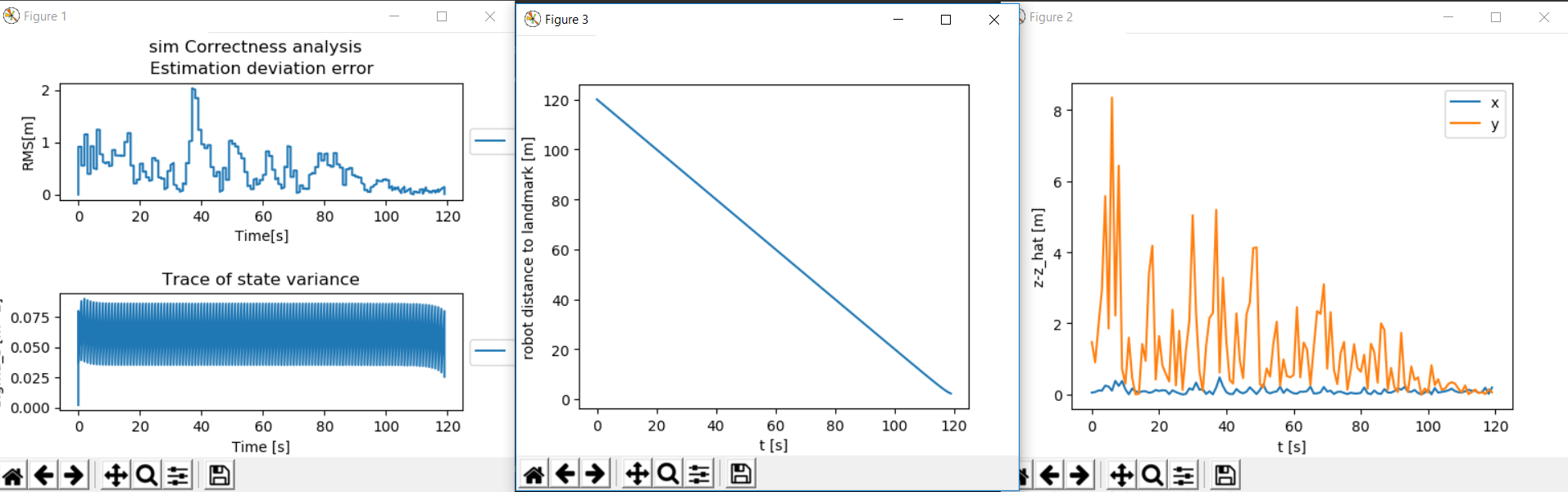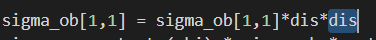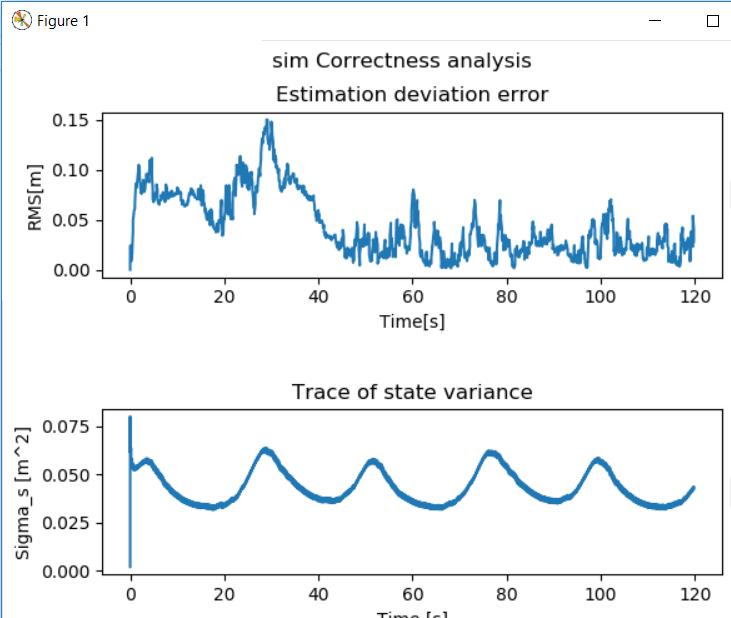11 Jul

#### RMS and trace Debugging

As part of the trace through of Colo-AT (primarily the Simulated Dataset Manager), one of the issues that arose was the proportionality of the RMS to measurement distance. In other words, as the distane between the robot and the landmark it was measuring increased the assosciated error in estimating the robots location also increased. The below two plots illustrate this effect as (z-ẑ) would grow and shrink as the robot moves farther and closer.

The straight line collection of plots corresponds to a robot moving towards a landmark. EKF_LS_CI.This plot corresponds to a robot moving a circle with a 360° FOV. And it moves closer and farther from the landmark. Centralized_EKFAfter some fixes, more intuitive behavior is observed. For example, the EKF_GS_CI2 algorithm (pre-implemented in Colo-AT) has a covariance matrix proportional to the measurement distance, while it's RMS is not proportional to distance. The code snippet and figure below illustrate this fact for a robot moving in a circle, towards and away from a fixed landmark.This means that the simulation confirms the implementation of the algorithm's update equations.